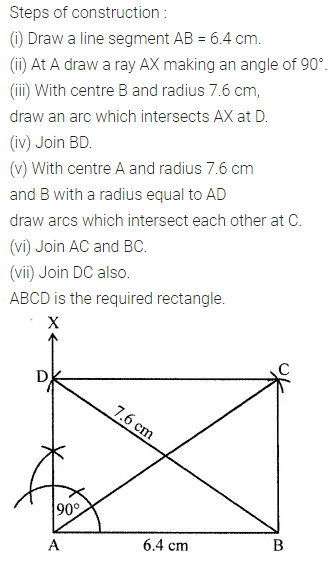## ML Aggarwal Class 8 Solutions for ICSE Maths Model Question Paper 5

Choose the correct answer from the given four options (1-2):
Question 1.
In a pentagonal pyramid number of faces, vertices and edges are
(a) F = 6, V = 6, E = 10
(b) F = 7, V = 7, E = 12
(c) F = 6, V = 8, E = 12
(d) none of these.
Solution: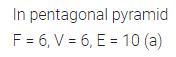Question 2.
If the lateral surface area of a cube is 144 cm, then its volume is
(a) 36cm3
(b) 216 cm3
(c) 288 cm3
(d) none of these
Solution: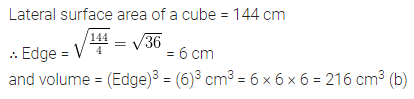Question 3.
Draw the top, front and side views of the given solid.Solution: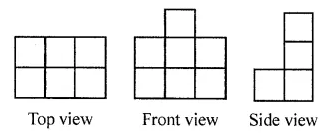Question 4.
The area of a trapezium is 96 cm2. If the lengths of its two parallel sides are 14 cm and 10 cm, then find the perpendicular distance between parallel sides.
Solution: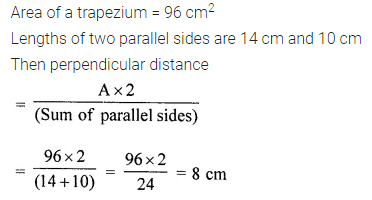Question 5.
In the given figure, C is the centre of the circle. The tangent to the circle of radius 6 cm from a point P outside the circle is of length 8 cm. Find the distance of the point P from the farthest point of the circumference.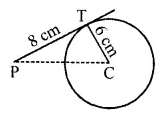Solution:Question 6.
Construct a quadrilateral PQRS in which PQ = 5.5 cm, RS = 6.5 cm, PS = 4.4 cm, PR = 6.5 cm and QS = 7.1 cm.
Solution: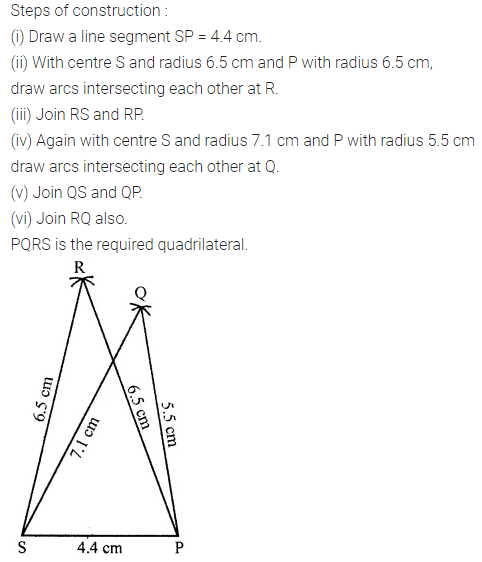Question 7.
The length, breadth and height of cuboidal water tank is 7 m, 6 m and 2 m respectively. If 4200 litre of water is pumped out from the tank. Find the fall in water level in the tank.
Solution: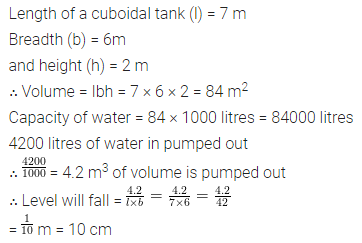Question 8.
Construct a rhombus PQRS such that PQ = 5.4 cm and ∠P = 60°. Also, draw its lines of symmetry.
Solution: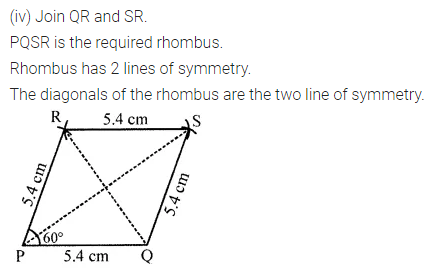Question 9.
A road roller has a diameter 84 cm and its width is 1.2 m. If it takes 500 complete revolutions to level a playground. Find the area of the playground. Also, find the cost of levelling the playground at a rate of 50 paise per m2.
Solution: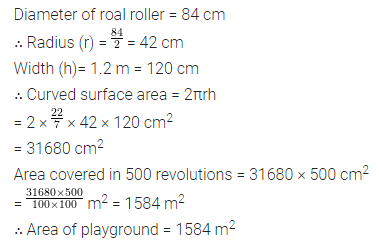Question 10.
Construct a rectangle ABCD, such that AB = 6.4 cm and BD = 7.6 cm.
Solution: